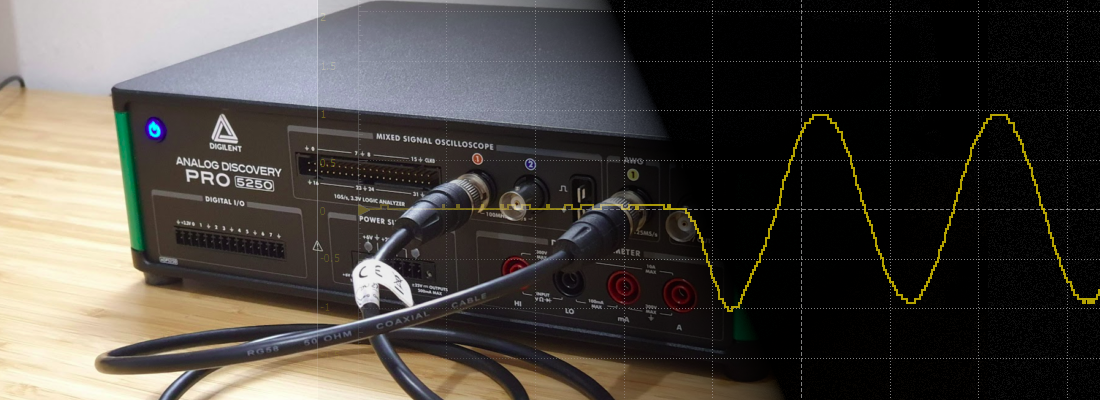When we is studying a degree related with electricity (electronics, electronics, electrical, communications, …), one of the elements which is very familiar to us is the oscilloscope. I remember when I started my electronics course (here in Spain we call it professional degree), we used analog oscilloscopes. This kind of oscilloscopes uses a cathodic ray tube, where a ray of electrons print in the phosphor screen the shape of the waveform. The direction of the ray are managed by 4 magnets, two of them move the ray in the horizontal axes (time axes), and the other two magnets move the ray in the vertical axis. All the elements of this oscilloscopes are analog, so the speed at they can move the ray in the horizontal axis will determine the maximum frequency that that can print. Also, in the vertical axis, the minimum amount of voltage that did the ray move, would be the voltage precision.

Times changes, and the way that we see the signals in the oscilloscope also has change. Nowadays, we have cheap oscilloscopes with better features than an expensive old oscilloscope, so, where is the catch? Now, we use digital oscilloscopes.

Digital oscilloscopes are acquisition systems where the signal, instead of be used in some algorithm, the signal is printed in a screen, more precisely, in a digital screen. Since digital oscilloscopes are digital acquisition systems, we have to deal with all the problems, and benefits, of the digital signal processing.

First of all, we have to deal with the sampling frequency. Remember the Nyquist criteria, we always need at least twice of the frequency to acquire a signal without a loss of quality. In other case we will obtain an alias of the original signals. Then, we need to quantify the signal, so we will print a signal with a worst SNR due to the acquisition noise (this is a very good article from Analog that talk about it). Besides all the loss due to the digital system, we will have an analog circuit before the digital acquisition, so we need to add the non-linearity, bias error, gain error… of the analog circuit. Until now, seems that we have step down in terms of features, but nothing could be further than the truth!, using digital oscilloscopes we have small oscilloscopes, even with very good features. Also, they has all the potential of its internal processors to execute in real time many DSP algorithm like filtering, FFT, peak detection… All of this makes possible more sophisticated triggers. Besides that, few years ago, the USB oscilloscopes arrived. Small oscilloscopes without screen that are connected to a computer, and uses a software to connect with the oscilloscope and show the waves.

To explore some of the features of the digital oscilloscopes, I am going to use the new ADP5250 from Digilent. To make some tests, I have used the integrated Arbitrary Waveform Generator (AWG) connected to the channel 1 of the oscilloscope.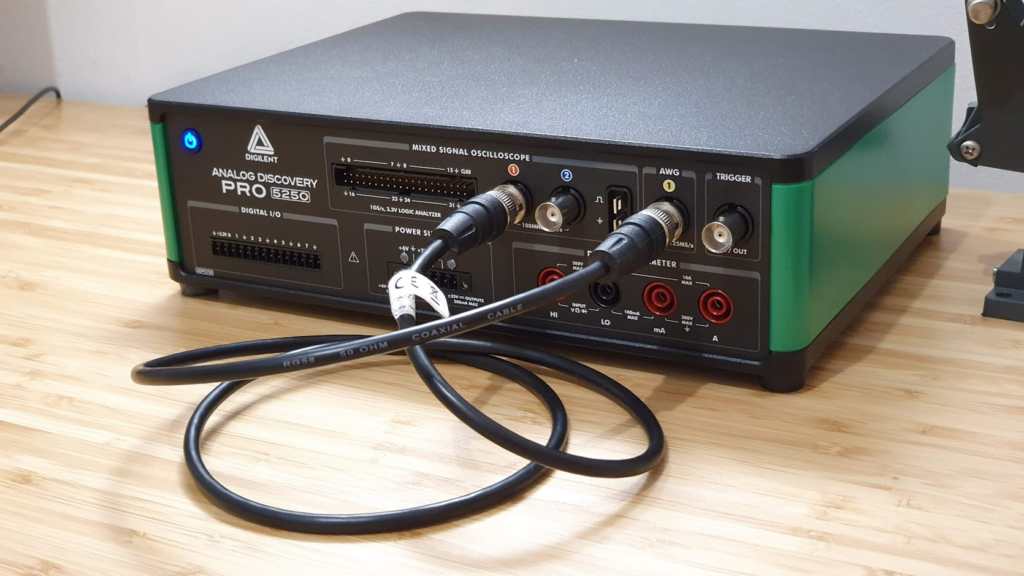Let’s take a look to its specifications.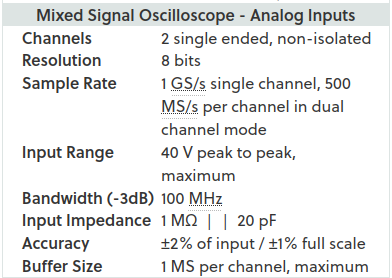The ADP5250 is a two channel, non isolated oscilloscope. This means that the negative terminal of both channels is connected, so if we connect each negative to different parts of the circuit, we have to be sure that they can be connected to each other.

Continuing with its features, next we have the resolution of the ADC that the oscilloscope uses to acquire the signal. In this case, it is based on a 8 bits ADC, so we have +-128 steps to represent the signal. The importance of this parameter is relative, since the gain of the input can be modified. The next image shows 2 different configurations for each channel, the configuration for the channel one can represent up to +-500 uV (the screen has five divisions), so the resolution is 500uV/128 = 3.9 uV (theoretically, because the features does not give us information about the SNR or SINAD). For the second channel, we can represent up to +- 1V , so the resolution is 1V/128 = 7.8 mV.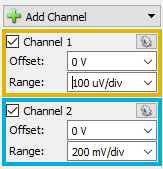Next is the most interesting part of an oscilloscope, its sampling rate, which is not the same as bandwidth, but they are related. The ADP5250 can achieve a sampling rate of 1 Gsps for single channel, and 0.5 Gsps in dual channel mode. This means that the oscilloscope only has an ADC of 1 Gsps and a resolution of 8 bits, and this ADC is shared between both channels. Furthermore, in the oscilloscope characteristics, we can find that the oscilloscope bandwidth is 100 MHz, a frequency at which it has an attenuation of 3 dB. Therefore, we have at the input a low pass filter, with a cutoff frequency of 100 MHz, followed by an ADC of ten times the sampling frequency, so aliasing will be almost eliminated. In the next image you can find the diagram of an oscilloscope similar to the ADP5250.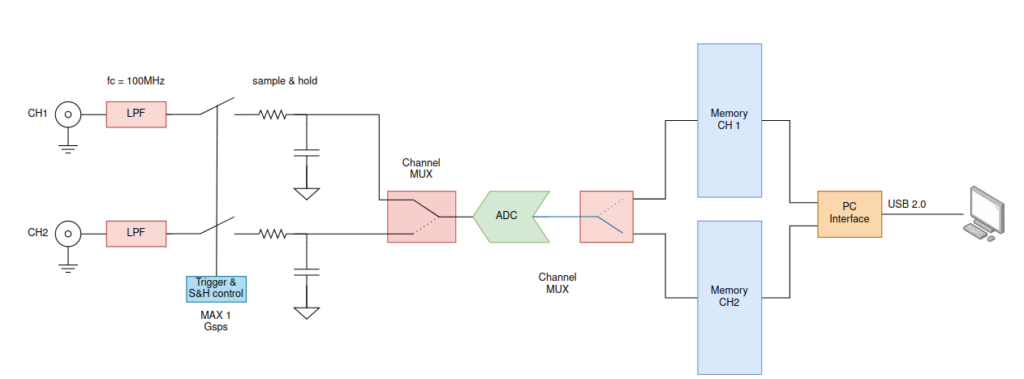In the diagram we can see the input, followed by the low pass filter and then the sample and hold circuit. This circuit must be duplicated in both channels since, in the case that we are using both channels, we want to see in the screen the value of both signals captured at the same time, so, even if we have only one ADC, we can store the analog value of both channels at the same time. Then, once the sample and hold capacitor is charged, the conversion is made one after another. Finally, the output of the ADC is stored in a memory.

Now, let’s talk about the memory, one of the strong points of this oscilloscope. The scope has 2 different memories, one for each channel. Each memory can store up to 1 million samples. This storage will allow capture large transitions at a high sampling rate. For example, if we are acquiring at 100 Msps, we can store up to 100 ms. Obviously, all the points don’t have to fit in the screen, but once acquired, we can apply a zoom in order to see the detail.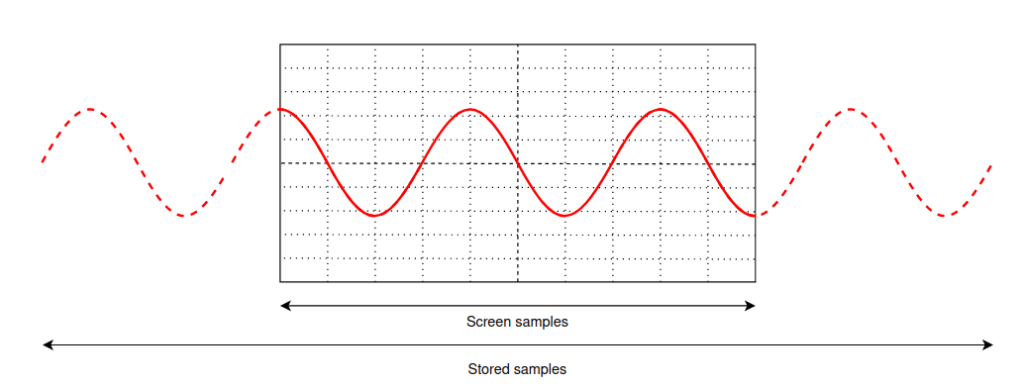The large number of samples that the ADP5250 can store, allow us to take captures like the next. In this capture, the power up of the waveform generator is captured. The entire capture has a duration of 1ms, and the frequency of the waveform is 10 MHz. The entire capture is shown in the next figure.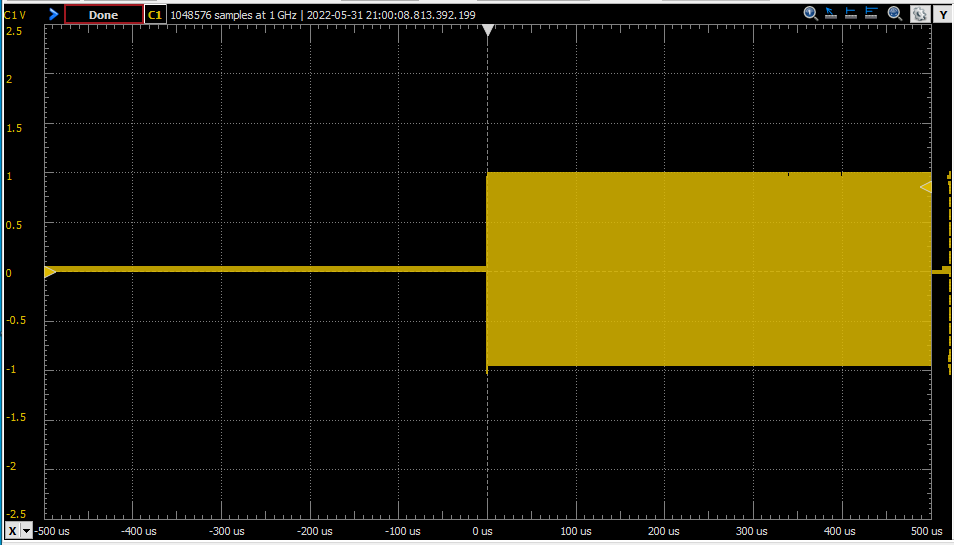Increasing the zoom of the signal, in the same capture, we can see the sine waveform.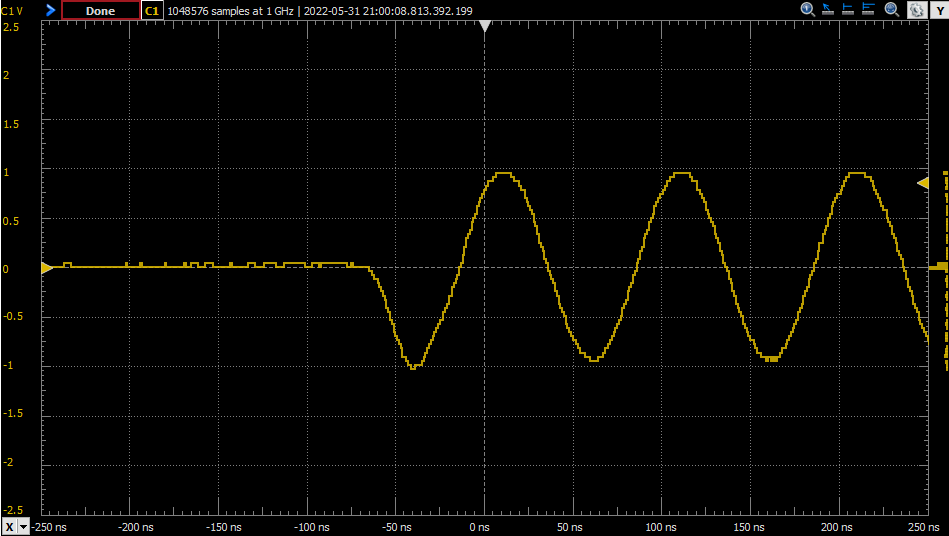Another advantage of the large amount of stored points is the resolution of the FFT. If we take a look to our college books about the DFT and the FFT, the frequency resolution is

$f_{res}=\frac{fsample}{2 \cdot nsamples}$

The value of two in the denominator is due to the fact that the FFT computed by Waveforms, only shows the half of the sampling frequency.

So if we have a large number of samples, we will detect more harmonics. In the next image, I have generated a sine signal with a frequency of 10 Mhz, and the sampling frequency fixed to 50 MHz. The number of samples is 64k.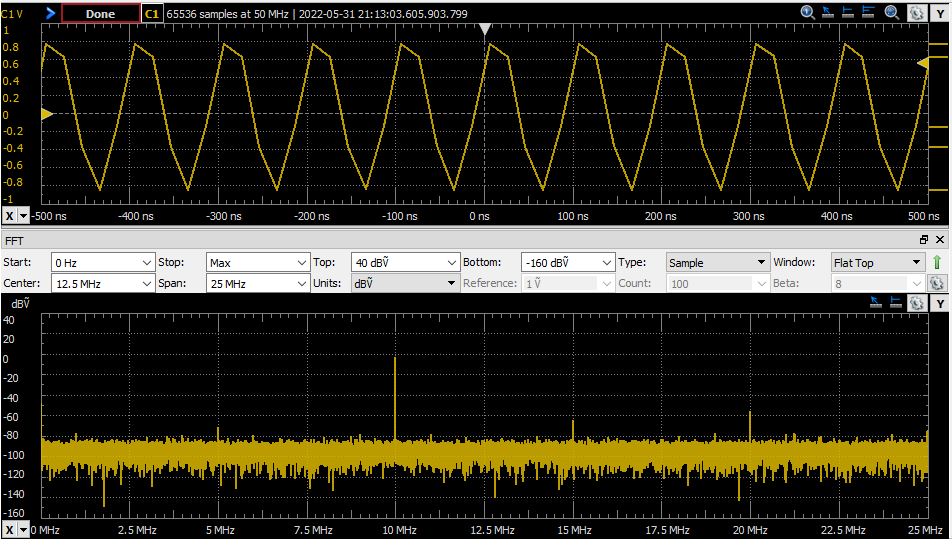Then, the same signal, with the same sampling rate, but using only 4k points.We can see how, although the temporal signal is the same, the resolution, and the width of the tone that represents the main harmonic is better when the number of points is larger.

Oscilloscopes are tools, like a hammer, and you need to know what are you doing when use them, like using a hammer… Digital oscilloscopes has lights and shadows, and in order to see the signal that you are acquiring, you need to know some basics concepts like sampling frequency, resolution, aliasing… but they are concepts that you sure learn in your degree or even at school. The lights of these oscilloscopes are many, size, real time processing, PC connection, and specially, price. Is not hard to find cheap oscilloscopes with reasonable features, and this makes than many hobbyist could buy one, and experiment with electronics. Furthermore, oscilloscopes like the ADP5250, that are connected through the USB to the computer, make easy to take captures of the waveform, process them using Python or MATLAB, and store them to include them into a document, or a blog post…